# Exploring Limits and Continuity

## Introduction

The limit of a function is a fundamental concept in calculus and analysis concerning the behaviour of that function near a particular input.
In this activity we will use the commands syms and limit to calculate limits of real functions. Furthermore, we will learn how to plot piecewise functions using the commands piecewise and fplot.

## Before starting

Use the MATLAB Live Editor to edit and run this Live Script in your browser or your desktop.
2. Run the code within a section before starting to read the next.
3. To run the code from each section, position the cursor on the code with the mouse and then click on the Run Section button (from the toolstrip) or click on the blue stripe on left side of that section as shown below: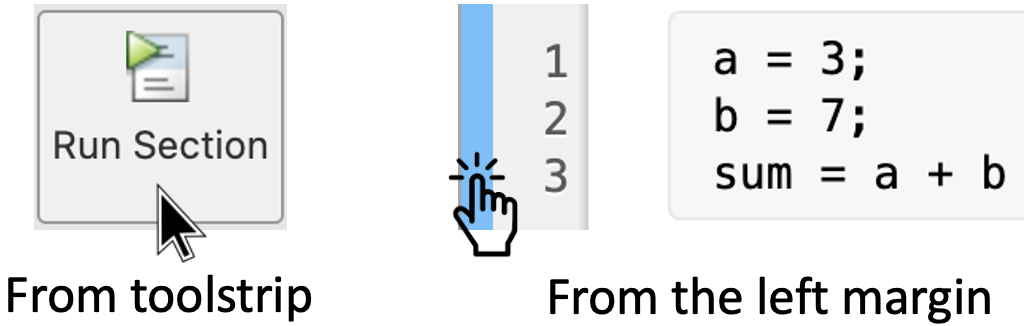Remark: Run the code of each section from top to bottom, otherwise you may get an error.
- The end of a section is indicated with a thin line, like the next one -

## 1. Calculating limits

### 1.1 Basic form of limit

MATLAB provides the limit command for calculating limits. In its most basic form, the limit command takes an expression as an argument and finds the limit of this expression as the independent variable goes to zero.
For example, let us calculate the limitThe limit command falls in the realm of symbolic computing; you need to use the syms command to tell MATLAB which symbolic variables you are using. That is:
syms x % Define symbolic variable
limit((x^3 + 5)/(x^4 + 7)) % Calculate limit

### 1.2. Limit of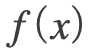when x tends to a

You can also compute limit of a function, as the variable tends to some number other than zero. In general, to calculatewe use the command limit(f, x, a) where "f" is the expression, "x" is the variable and "a" is the number that "x" is approaching.
For example, let us calculate:
•; and
•.
Thus, we write:
syms x % Define symbolic variable "x"
limit(x^2 + 5, x, 3)
limit((2 * x - 3)/(x - 1), x, 1)
Remark: If the f depends on only one variable, we can omit it in the code, e.g. limit(x^2 + 5, 3).
Also notice that the output of limit((2 * x - 3/(x - 1)) is NaN (not a number). This means that the limit does not exist! We can appreciate this by analysing the plot the graph ofusing the command fplot as follows:
syms x % Define symbolic variable "x"
g0 = (2 * x - 3)/(x - 1); % Define a variable "g0" in terms "x"
fplot(x, g0) % Plot the function
xlabel('x') % Now we just add some settings to the plot
ylabel('g(x)=(2x-3)/(x-1)')
grid on
axis([-4 4 -8 8])
Run this section and analyse the graph of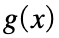. What do you notice?
Another way to confirm that the limit does not exist is by calculating one-sided limits.

### 1.3 One-sided limits

We can also calculate the left-handed and right-handed limits. These are computed in MATLAB by passing the character strings 'left' and 'right' to the limit command as the last argument. For example:
syms x
g0 = (2 * x - 3)/(x - 1)
limit(g0, x, 1,'left')
limit(g0, x, 1,'right')
Remark: In this case we cannot omit the variable "x" in the command limit. Try it yourself! Delete "x" in any of the lines with the limit commad, from the previous code, and re-run this section. You will get an error because MATLAB always requires the symbolic variable to calculate one-sided limits.

#### 1.3.1 Piecewise functions

In MATLAB we can also calculate limits of piecewise functions. For example, consider the functionBefore calculating limits, first let us plot this function on the interval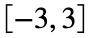using the commands piecewise and fplot as follows:
syms x
f0 = piecewise(x < 0, 3/2 * x - 1, 0 <= x, exp(x^2)/(1 + x^2))
fplot(f0,'r')
grid on, xlabel('x'), ylabel('y'), axis([-3,3,-4,4])
Remark: Notice that all the commands in the last line of the previous code are separated with a comma ",". Compare with the code for plotting the example from section 1.2.
Now we can calculate the one-sided limits of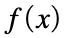. To do this we can make use of the value of the variable f0, defined above. Thus, we have:
syms x
limit(f0, x, 0, 'left')
limit(f0, x, 0, 'right')
Questions: Do these values agree with the graph previously plotted? Is this function continuous or discontinuous at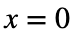?

### 1.4 Limits to Infinity

MATLAB allows you also to calculate limits approaching to infinity. For example, in order to calculate
••we just need to write:
syms x
func = (2 * x - 3)/(x - 1)
limit(func, x, Inf)
limit(func, x, -Inf)
Note: You can confirm the results from the plot of section 1.2.

### 1.5 Verification of Basic Properties of Limits

We can also verify the basic properties of limits using the command limit. For example, let us calculate the limit of the functions
••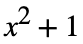as x tends to 5. To do this we write:
syms x
f = (3 * x - 5)/(x - 3);
g = x^2 + 1;
l1 = limit(f, 4)
l2 = limit (g, 4)
lAdd = limit(f + g, 4)
lSub = limit(f - g, 4)
lMult = limit(f * g, 4)
lDiv = limit (f / g, 4)

## 2. Hands on practice

Let's practice what we just learned.

### Activity 1:

Calculate the following limits:
1.2.3.4.### Activity 2:

#### Part 1 - Plotting piecewise functions

Use the commands piecewise and fplot to plot the graphs of the following functions on the indicated axes.
a), with the x-axis raging from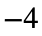to 4 and the y-axis ranging from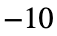to 8.
b), with the x-axis raging from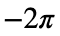to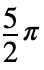and the y-axis ranging from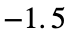to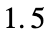.
Suggestion: Remember to use different variables in your code. You can add labels to identify each graph and change the axis scales.
Write your code forhere:
Write your code forhere:

#### Part 2 - Are these functions continuous or discontinuous?

In section 1.3.1 we discussed how to calculate one-sided limits of piecewise functions.
Determine ifis continuous or discontinuous at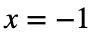. Write your code here:
Determine ifis continuous or discontinuous at. Write your code here: